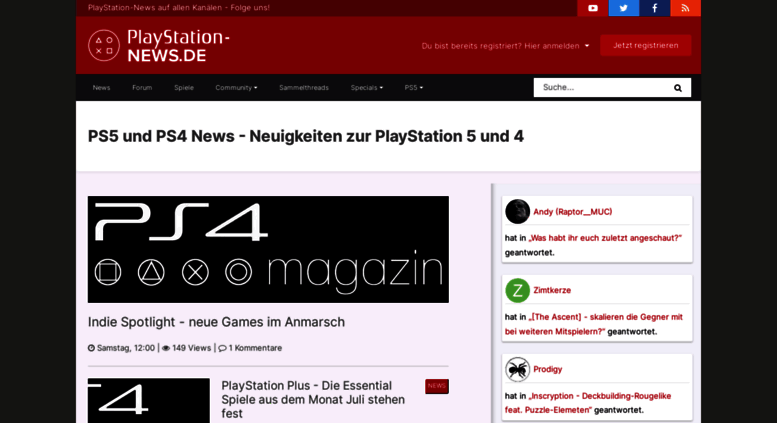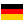# ps4-magazin.de: PS5 News & PS4 News und alle Infos zur PlayStaion 5

PS5 News & PS4 News. Das große PS5 & PS4 Forum. Das alles auf PlayStation-News.de!

• 53/100

Normal result
• 5

Successful tests
• 3

Failed tests
• First response

295 ms

5.2 sec

• Page rendered

694 ms

6.2 sec

Click here to check amazing PS4 Magazin content for Germany. Otherwise, check out these important facts you probably never knew about ps4-magazin.de

We analyzed Ps4-magazin.de page load time and found that the first response time was 295 ms and then it took 5.9 sec to load all DOM resources and completely render a web page. This is a poor result, as 75% of websites can load faster.

## Page optimization

•  HTML 148.7 kB Images 837.8 kB Javascripts 1.0 MB CSS 118.6 kB

In fact, the total size of Ps4-magazin.de main page is 2.1 MB. This result falls beyond the top 1M of websites and identifies a large and not optimized web page that may take ages to load. 80% of websites need less resources to load and that’s why Accessify’s recommendations for optimization and resource minification can be helpful for this project. Javascripts take 1.0 MB which makes up the majority of the site volume.

• Original 148.7 kB
• After minification 129.6 kB
• After compression 29.4 kB

HTML optimization

HTML content can be minified and compressed by a website’s server. The most efficient way is to compress content using GZIP which reduces data amount travelling through the network between server and browser. This page needs HTML code to be minified as it can gain 19.1 kB, which is 13% of the original size. It is highly recommended that content of this web page should be compressed using GZIP, as it can save up to 119.3 kB or 80% of the original size.

• Original 837.8 kB
• After optimization 767.6 kB

Image optimization

Image size optimization can help to speed up a website loading time. The chart above shows the difference between the size before and after optimization. PS4 Magazin images are well optimized though.

• Original 1.0 MB
• After minification 980.0 kB
• After compression 325.7 kB

JavaScript optimization

It’s better to minify JavaScript in order to improve website performance. The diagram shows the current total size of all JavaScript files against the prospective JavaScript size after its minification and compression. It is highly recommended that all JavaScript files should be compressed and minified as it can save up to 682.3 kB or 68% of the original size.

• Original 118.6 kB
• After minification 102.7 kB
• After compression 22.7 kB

CSS optimization

CSS files minification is very important to reduce a web page rendering time. The faster CSS files can load, the earlier a page can be rendered. Ps4-magazin.de needs all CSS files to be minified and compressed as it can save up to 95.8 kB or 81% of the original size.

### Network requests diagram

• ps4-magazin.de
• www.ps4-magazin.de
• protoaculous.js
• default.js
• AjaxRequest.class.js
• burningBoard.css
• style-3.css
• jquery.min.js
• jquery.socialshareprivacy.js
• p4m.js
• iam.js
• mfront.css
• p4m.css
• network.css
• analytics.js
• bg.jpg
• orange_white_40-94fc761.png
• gplus-64.png
• sitebg.png
• logo.png
• android_app.png
• searchSubmitS.png
• logo_clean.png
• avatar-9206.jpg
• avatar-5882.jpg
• avatar-38.jpg
• avatar-9067.gif
• avatar-9717.jpg
• avatar-9770.jpg
• avatar-default.png
• avatar-9703.jpg
• avatar-9433.png
• avatar-9766.jpg
• avatar-9414.jpg
• twitch.png
• 495-200.jpg
• 587-200.jpg
• 585-200.jpg
• 314-200.jpg
• 354-200.jpg
• gamedb_default_packshot.jpg
• helpS.png
• legalNoticeS.png
• termsOfUseS.png
• contactS.png
• styleOptionsS.png
• dateS.png
• Netzwerk2.png
• qs.ioam.de
• tx.io
• upS.png
• collect
• onlinewelten.js
• submitS.png
• globalV6.js
• rc
• c.ount
• gw.js
• pql
• tx.io
• 519756287.js
• pixel
• pixel
• default.js
• oba_icon.png
• 185ff1dd14fd529ecfcc833e414d76e
• 35136,82960,35132,35134,82959,35138
• p.min.js
• data
• c.ount
• www.ps4-magazin.de;fv=0;sx=1680;sy=1050;zz=1x1;site=DE_OW.ps4-magazin;zone=other;idf=onlinewelten;c1=0;c2=0;c3=0;c4=1;c5=1;c6=0;c7=0;c8=0;c9=0;c10=0;c11=1;c12=0;c13=0;c14=0;c15=0;c16=1;c17=0;c19=0;c20=0;d1=1;d3=5;d4=0;d8=1;d9=3;d10=1;d12=1;d15=3;d16=1;d17=1;d20=1;d21=0;d22=-1;i55=0;i3=1;i1=1;i13=1;i21=1;i28=1;i42=1;i46=1;i53=1;n3=1;n5=1;n8=1;n9=0;f1=1;f2=1;f3=1;xx=pl;xx=fa;xx=pb;xx=pd;xx=bb;xx=wp;xx=fp;xx=hp;xx=sb;xx=pu1;xx=fb2;xx=rt1;xx=sc1;KW=pc,fantasy,rpg,scifi,mmo,fantasy,action,multiplayer,game;!c=ga;tile=1;ord=3025018156
• rs.js
• rpc.flow
• vce_st.js
• www.ps4-magazin.de;fv=0;sx=1680;sy=1050;zz=468x60;site=DE_OW.ps4-magazin;zone=other;idf=onlinewelten;c1=0;c2=0;c3=0;c4=1;c5=1;c6=0;c7=0;c8=0;c9=0;c10=0;c11=1;c12=0;c13=0;c14=0;c15=0;c16=1;c17=0;c19=0;c20=0;d1=1;d3=5;d4=0;d8=1;d9=3;d10=1;d12=1;d15=3;d16=1;d17=1;d20=1;d21=0;d22=-1;i55=0;i3=1;i1=1;i13=1;i21=1;i28=1;i42=1;i46=1;i53=1;n3=1;n5=1;n8=1;n9=0;f1=1;f2=1;f3=1;xx=pl;xx=fa;xx=pb;xx=pd;xx=bb;xx=wp;xx=fp;xx=hp;xx=sb;xx=pu1;xx=fb2;xx=rt1;xx=sc1;KW=pc,fantasy,rpg,scifi,mmo,fantasy,action,multiplayer,game;!c=ga;tile=2;ord=3025018156
• c.ount
• p2
• p
• p
• rpc.flow
• www.ps4-magazin.de;fv=0;sx=1680;sy=1050;zz=160x600;site=DE_OW.ps4-magazin;zone=other;idf=onlinewelten;c1=0;c2=0;c3=0;c4=1;c5=1;c6=0;c7=0;c8=0;c9=0;c10=0;c11=1;c12=0;c13=0;c14=0;c15=0;c16=1;c17=0;c19=0;c20=0;d1=1;d3=5;d4=0;d8=1;d9=3;d10=1;d12=1;d15=3;d16=1;d17=1;d20=1;d21=0;d22=-1;i55=0;i3=1;i1=1;i13=1;i21=1;i28=1;i42=1;i46=1;i53=1;n3=1;n5=1;n8=1;n9=0;f1=1;f2=1;f3=1;xx=pl;xx=fa;xx=pb;xx=pd;xx=bb;xx=wp;xx=fp;xx=hp;xx=sb;xx=pu1;xx=fb2;xx=rt1;xx=sc1;KW=pc,fantasy,rpg,scifi,mmo,fantasy,action,multiplayer,game;!c=ga;tile=3;ord=3025018156
• c.ount
• p
• rpc.flow
• search.png
• www.ps4-magazin.de;fv=0;sx=1680;sy=1050;zz=300x250;site=DE_OW.ps4-magazin;zone=other;idf=onlinewelten;c1=0;c2=0;c3=0;c4=1;c5=1;c6=0;c7=0;c8=0;c9=0;c10=0;c11=1;c12=0;c13=0;c14=0;c15=0;c16=1;c17=0;c19=0;c20=0;d1=1;d3=5;d4=0;d8=1;d9=3;d10=1;d12=1;d15=3;d16=1;d17=1;d20=1;d21=0;d22=-1;i55=0;i3=1;i1=1;i13=1;i21=1;i28=1;i42=1;i46=1;i53=1;n3=1;n5=1;n8=1;n9=0;f1=1;f2=1;f3=1;xx=pl;xx=fa;xx=pb;xx=pd;xx=bb;xx=wp;xx=fp;xx=hp;xx=sb;xx=pu1;xx=fb2;xx=rt1;xx=sc1;KW=pc,fantasy,rpg,scifi,mmo,fantasy,action,multiplayer,game;!c=ga;tile=4;ord=3025018156
• c.ount
• express_html_inpage_rendering_lib_200_121.js
• 1x1.jpg
• pixel
• generic
• p
• rpc.flow
• ca-pub-8891272111492592.js
• zrt_lookup.html
• newstypebg.png
• generic
• match
• mtrcs_380420.js
• lidar.js
• index.html
• match
• match
• TweenMax.min.js
• Physics2DPlugin.min.js
• TKUT_v1.1.1.min.js
• sparkle_pink.png
• sparkle_white.png
• bg.jpg
• star.png
• logo.png
• txt1.png
• txt2.png
• txt3.png
• txt4.png
• cta.png
• gfx_white.png
• all.js
• 495-738.jpg
• match
• match
• activeview
• osd.js
• track.php
• 2.gif
• 2472579636139788460
• abg.js
• m_js_controller.js
• mtrcs_380420.js
• t.js
• xd_arbiter.php
• xd_arbiter.php
• submit
• s
• x_button_blue2.png
• print.css
• 1.gif
• analytic.js
• p.skimresources.com
• E4WYPs_lNJIuXrWpTsOcc3FIG36RfI0ERc0srw5QW9Y.js
• submit
• pixel
• cm.gif
• 2.gif
• p
• light-bottom.css
• ping
• logo.png
• like_box.php
• roNeOY-tz5Y.css
• UHhaxo8Cs3k.css
• dbNRhrdsQao.css
• 1ssvpqNRk1L.css
• O8DK6hTywHD.css
• Qz9nHTUX1Wv.css
• _rx8naC75Dy.js
• ZFt4Xru0ZkB.js
• ICDbTSzjQEg.js
• TTCre3391I0.js
• njO1TPvGzoR.js
• b_6HNn_J2BZ.js
• 7cm7D75Y0Sf.js
• rFmE1g6Dkoz.js
• 336JejShbZp.js
• C-h3nYPqETO.js
• oRoBXlHCpEy.js
• sj-JwAJi4AH.js
• DKRU1bYTkTw.js
• W0OV23mIOyO.js
• 36TdwhIHusi.js
• 998534_550819391620709_1926299028_n.jpg
• safe_image.php
• safe_image.php
• safe_image.php
• safe_image.php
• safe_image.php
• 1384318_590803840955597_119930497_n.jpg
• 12346382_476652655850759_3786353697602790616_n.jpg
• 10446641_436646216518380_7705019513233094386_n.jpg
• 11751849_1646286118942006_8661182269679527330_n.jpg
• 10334436_1053157871414450_4251579239596335446_n.jpg
• 12805798_967127063379634_8896798540838928561_n.jpg
• 12308237_1659021691005473_376307482235895263_n.jpg
• 10175001_115179688858103_8724277232315722013_n.jpg
• 12507198_1741299742756516_2766359095554987605_n.jpg
• wL6VQj7Ab77.png
• s7jcwEQH7Sx.png
• QDwYpIaRyfG.png
• a-ZN6WoEOje.png
• pUFVdPjIRct.png
• I6-MnjEovm5.js
• vlXaquuXMrr.js
• ui

Our browser made a total of 227 requests to load all elements on the main page. We found that 26% of them (59 requests) were addressed to the original Ps4-magazin.de, 13% (29 requests) were made to Static.xx.fbcdn.net and 8% (18 requests) were made to S0.2mdn.net. The less responsive or slowest element that took the longest time to load (835 ms) relates to the external source Static.xx.fbcdn.net.

Requests

The browser has sent 217 CSS, Javascripts, AJAX and image requests in order to completely render the main page of PS4 Magazin. We recommend that multiple CSS and JavaScript files should be merged into one by each type, as it can help reduce assets requests from 75 to 1 for JavaScripts and from 16 to 1 for CSS and as a result speed up the page load time.

75

Javascripts

114

Images

16

CSS

12

AJAX

217

All

Possible request optimization

1

Javascripts

70

Images

1

CSS

12

AJAX

133

Optimized

84

All

Normal result

Redirects

As for redirects, our browser was forwarded to http://www.ps4-magazin.de/ before it reached this domain.

Ps4-magazin.de uses IP addresses which are currently shared with 7 other domains. The more sites share the same stack of IP addresses, the higher the host server’s workload is. It is strongly recommended that the host server should be changed or the hosting provider should be requested to give a different (separate) IP address for this domain.

Normal result

IP Trace

ps4-magazin.de

series.vg

pzwiki.net

gogoaway.com

104.21.2.138

ps4-magazin.de

usic.org.ua

arizonasnowbowl.com

velocityreviews.com

pzwiki.net

172.67.129.71

DNS records

Type Host Target/ip TTL Other
A

ps4-magazin.de

104.21.2.138 300
A

ps4-magazin.de

172.67.129.71 300
NS

ps4-magazin.de

dana.ns.cloudflare.com 86400
NS

ps4-magazin.de

seth.ns.cloudflare.com 86400
SOA

ps4-magazin.de

3600 Mname: dana.ns.cloudflare.com
Rname: dns.cloudflare.com
Serial: 2035799239
Refresh: 10000
Retry: 2400
Expire: 604800
Minimum-ttl: 3600

Language and encoding

Good result

Language

DEDetected

DEClaimed

Encoding

UTF-8

Claimed

Language claimed in HTML meta tag should match the language actually used on the web page. Otherwise Ps4-magazin.de can be misinterpreted by Google and other search engines. Our service has detected that German is used on the page, and it matches the claimed language. Our system also found out that Ps4-magazin.de main page’s claimed encoding is utf-8. Use of this encoding format is the best practice as the main page visitors from all over the world won’t have any issues with symbol transcription.

Similarly rated websites

HTTPS certificate

SSL Certificate

Ps4-magazin.de has no SSL certificate. Web browsing can be safer with HTTPS connection, so we suggest that it should be obtained for this site.

Poor result

Visitor World Map

Country of origin for 99.8% of all visits is Germany. It lies approximately 4880 miles away from the server location (United States) and such a long distance can negatively affect website speed, as it takes some time for data to travel back and forth between those places. That’s why one of the best ways to speed up Ps4-magazin.de page load time for the majority of users is moving the server to Germany or just closer to the user base.

Poor result

Newly analyzed websites

Poor result

Social Sharing Optimization

Open Graph description is not detected on the main page of PS4 Magazin. Lack of Open Graph description can be counter-productive for their social media presence, as such a description allows converting a website homepage (or other pages) into good-looking, rich and well-structured posts, when it is being shared on Facebook and other social media. For example, adding the following code snippet into HTML <head> tag will help to represent this web page correctly in social networks:

ps4-magazin.de